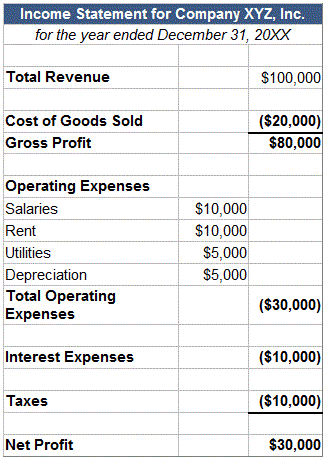Accounting

# Net Profit Margin: Mathematical Explanation

Net profit margin is the percentage of revenue remaining after all operating expenses, interest, taxes and preferred stock dividends (but not common stock dividends) have been deducted from a company’s total revenue.

Example:

The formula for net profit margin is:

(Total Revenue – Total Expenses)/Total Revenue = Net Profit/Total Revenue = NetProfit Margin

By dividing net profit by total revenue, we can see what percentage of revenue made it all the way to the bottom line, which is good for investors.

Let’s look at a hypothetical income statement for Company XYZ:Using the formula and the information above, we can calculate that Company XYZ’s net profit margin was \$30,000/\$100,000 = 30%

Why it Matters:

Net profit margin is one of the most closely followed numbers in finance. Shareholders look at net profit margin closely because it shows how good a company is at converting revenue into profits available for shareholders.

One of the most important concepts to understand is that net profit is not a measure of how much cash a company earned during a given period. This is because the income statement includes a lot of non-cash expenses such as depreciation and amortization. To learn about how much cash a company generates, you need to examine the cash flow statement.

Changes in net profit margin are endlessly scrutinized. In general, when a company’s net profit margin is declining over time, a myriad of problems could be to blame, ranging from decreasing sales to poor customer experience to inadequate expense management.

Net profit margin is often used to compare companies within the same industry, in a process known as “margin analysis.” Net profit margin is a percentage of sales, not an absolute number, so it can be extremely useful to compare net profit margins among a group of companies to see which are most effective at converting sales into profits.